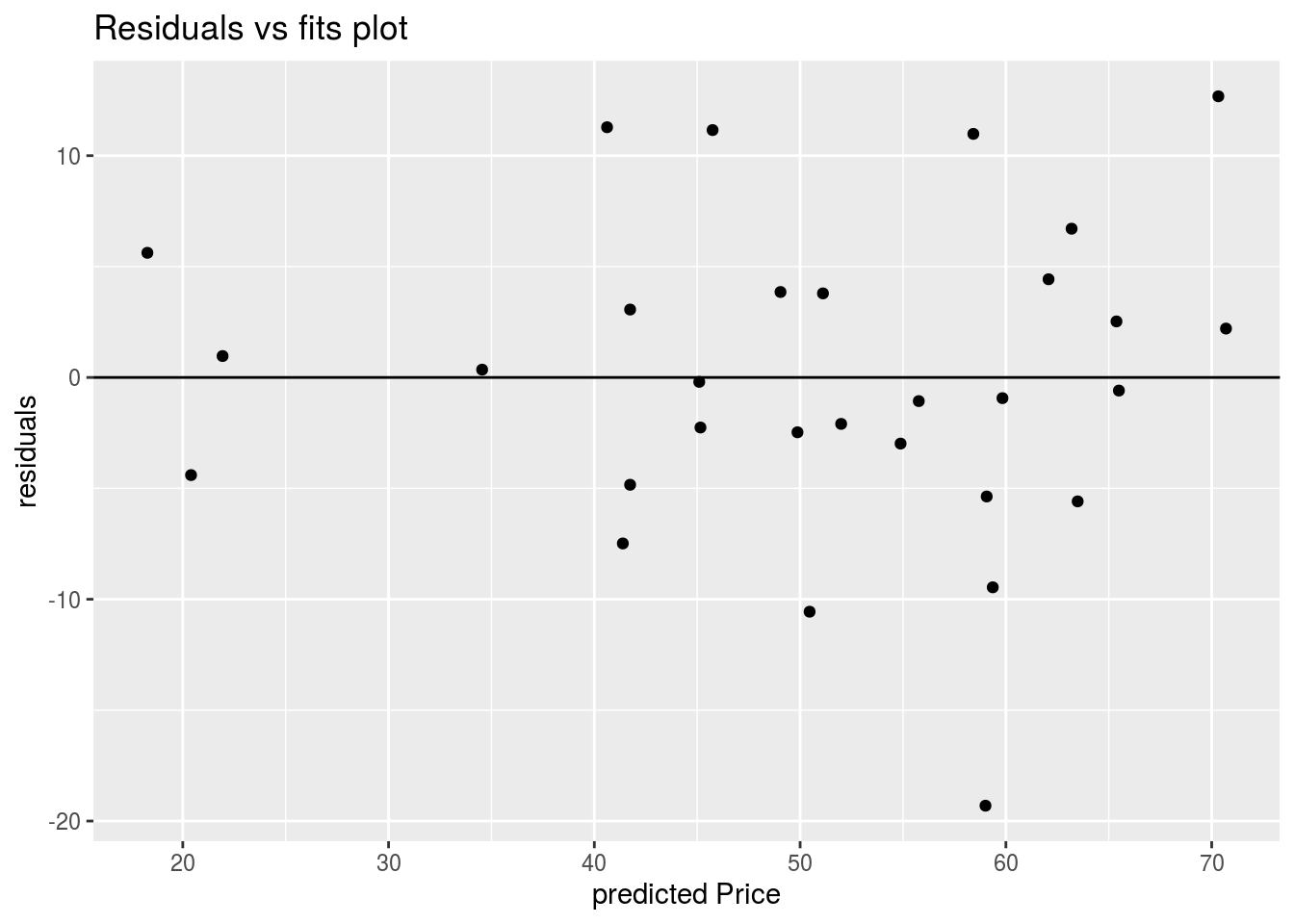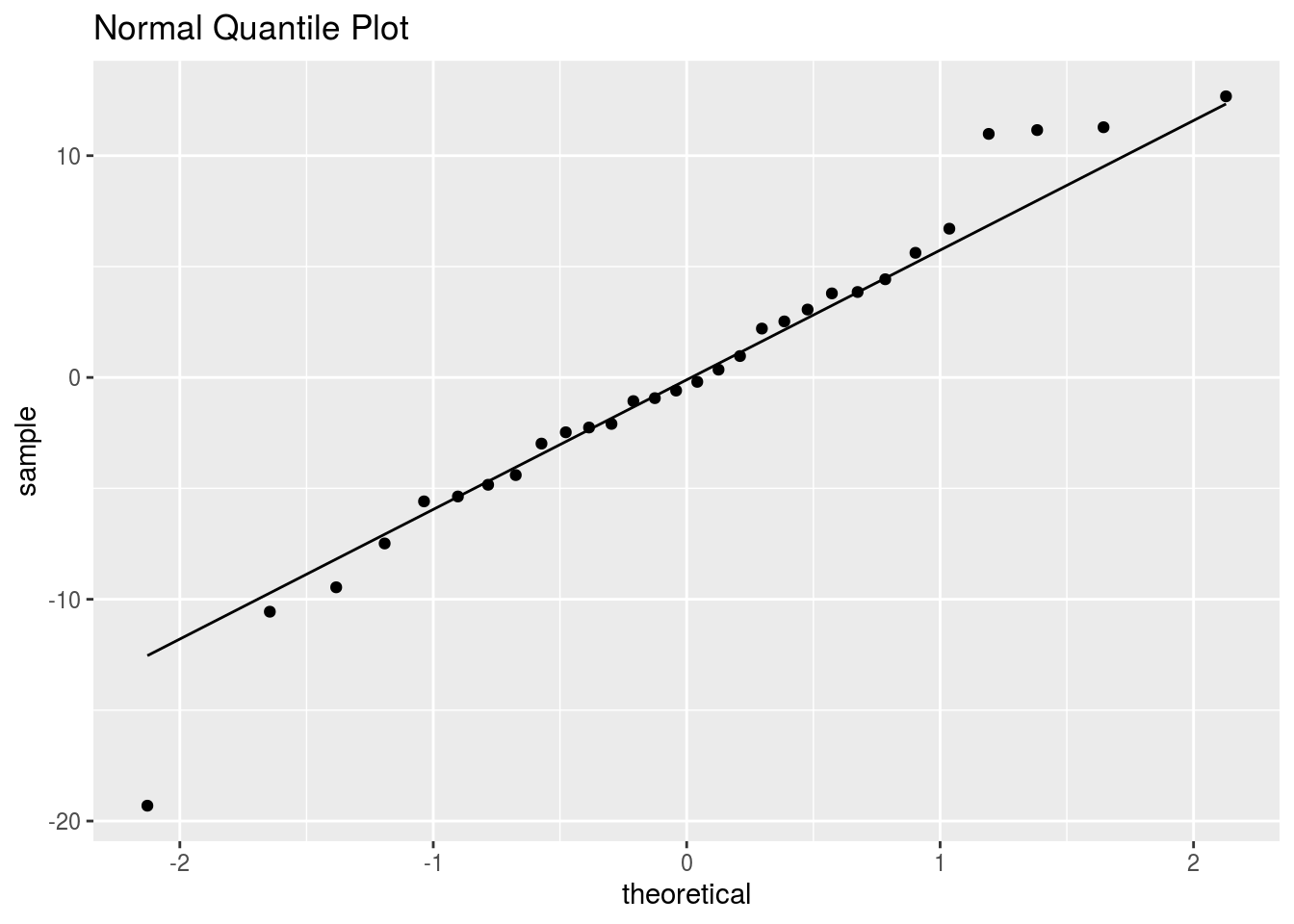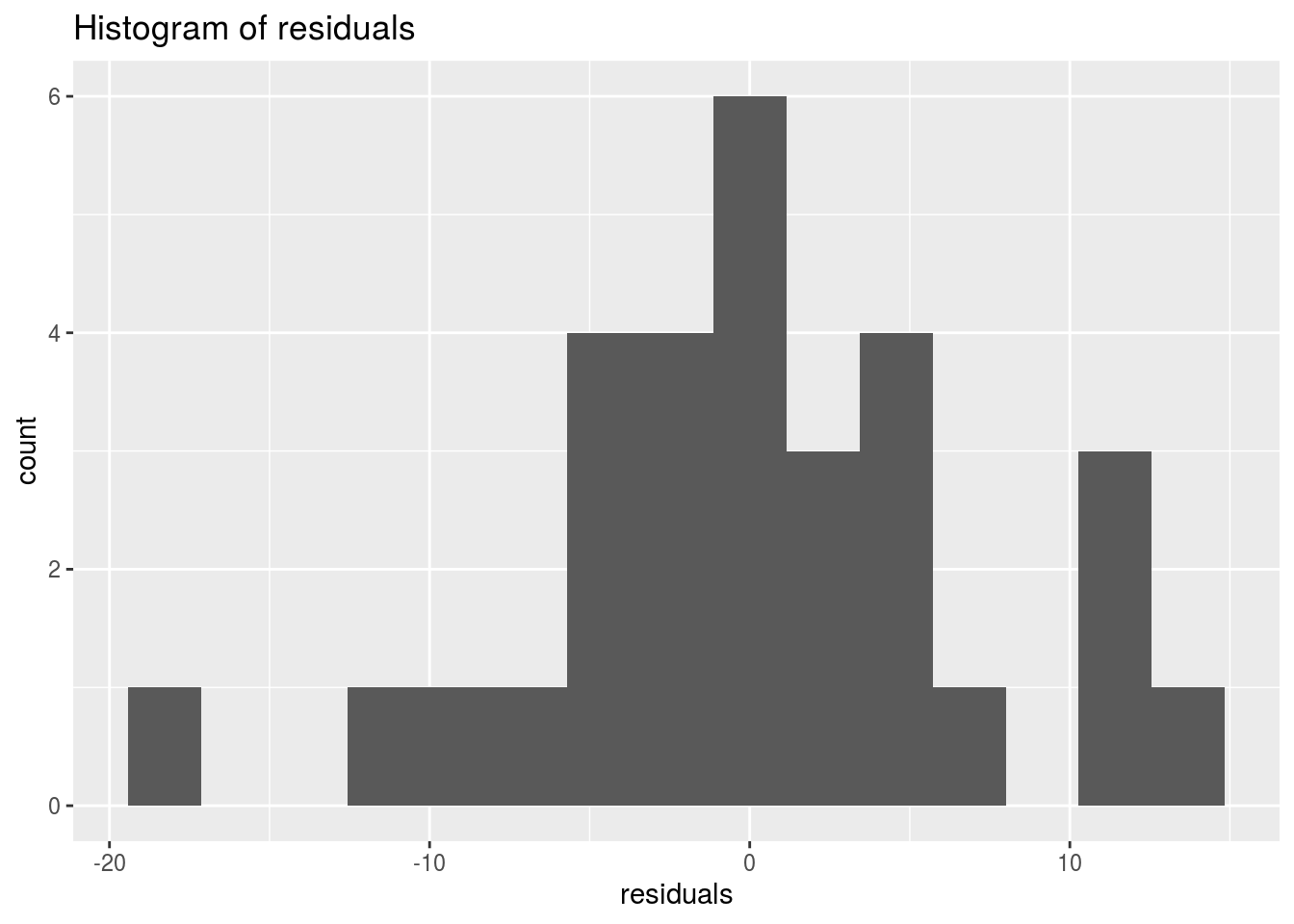library(tidyverse)
library(Stat2Data)
data("PorschePrice")

## Using the PorschePrice data, fit a linear model of Price ~ Mileage

lm(Price ~ Mileage, data = PorschePrice)
##
## Call:
## lm(formula = Price ~ Mileage, data = PorschePrice)
##
## Coefficients:
## (Intercept)      Mileage
##     71.0905      -0.5894

## Add a variable called y_hat to the PorschePrice dataset with the predicted y values

y_hat <- lm(Price ~ Mileage, data = PorschePrice) %>%
predict()

PorschePrice <- PorschePrice %>%
mutate(y_hat = y_hat)

## Add another variable called residuals to the PorschePrice dataset with the residuals

PorschePrice <- PorschePrice %>%
mutate(residuals = Price - y_hat)

## Create a plot that examines the “linearity” and “constant variance” assumptions.

ggplot(PorschePrice, aes(x = y_hat, y = residuals)) +
geom_point() +
geom_hline(yintercept = 0) +
labs(title = "Residuals vs fits plot",
x = "predicted Price")## Create a plot that examines the “normality” assumption

ggplot(PorschePrice, aes(sample = residuals)) +
geom_qq() +
geom_qq_line() +
labs(title = "Normal Quantile Plot")ggplot(PorschePrice, aes(residuals)) +
geom_histogram(bins = 15) +
labs(title = "Histogram of residuals")